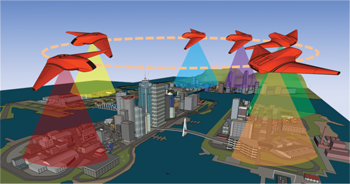# Highlight

## Monitoring of Stochastic EventsWe introduce a new mobile sensor scheduling problem involving a robot tasked to monitor several events occurring at spatially distributed locations. Of particular interest is the monitoring of transient events of stochastic nature, with applications ranging from natural phenomena (e.g., monitoring abnormal seismic activity around a volcano using a ground robot) to urban activities (e.g., monitoring early formations of traffic congestion using an aerial robot). Here, these stochastic arrival processes are modeled as an independent Poisson processes with various inter-arrival rates.

In monitoring such events, the robot seeks to: (i) maximize the number of events observed and (ii) minimize the delay between consecutive observations of events occurring at the same location. We are interested in finding a cyclic patrolling route that that optimizes these objectives in a balanced manner. To tackle the problem, first, assuming the cyclic ordering of stations is known, we prove the existence and uniqueness of the optimal solution, and show that the solution has desirable convergence rate and robustness. Our constructive proof also yields an efficient algorithm for computing the unique optimal solution with linear time complexity. The analysis remains valid when the cyclic order is unknown. We then provide a polynomial-time approximation scheme that computes for any ε > 0 a (1+ε)-optimal solution for the more general, NP-hard problem in which the order of the stations is unknown. Click below for more information.

Optimal Patrolling Policy for Persistent Monitoring of Stochastic Events by Professor Jingjin Yu